Geometry

# Composite Perimeters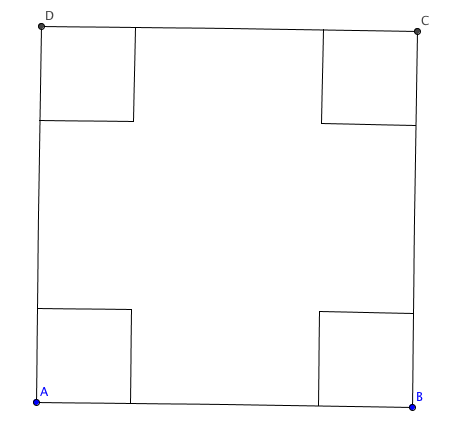Starting with a square piece of paper that measures 20 inches by 20 inches, Calvin cuts out a 5 inch by 5 inch square from each of the 4 corners. What is the perimeter of the resulting shape?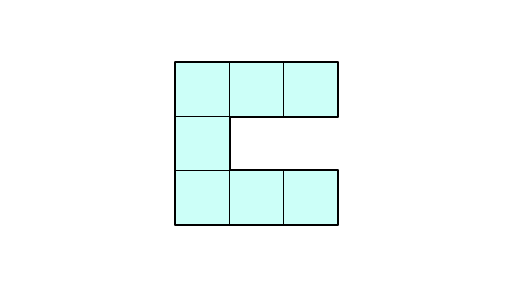The shape above is made of 7 squares. If the side length of a square is 3, what is the perimeter of the shape?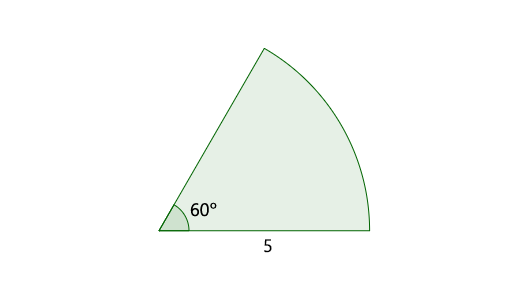If the radius of the sector above is $5$ and its central angle is $60^\circ,$ what is its perimeter?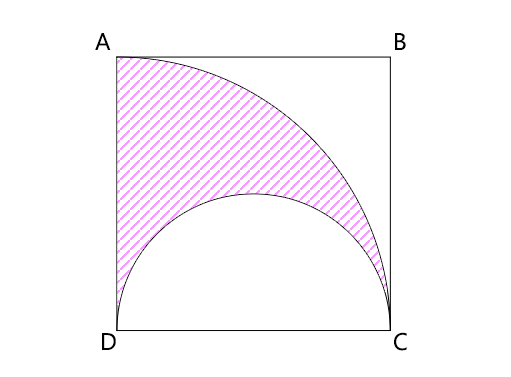$\square{ABCD}$ is a square, and the arcs are of a semi-circle and a quarter-circle. If $\overline{AB}=2$, what is the perimeter of the colored region above?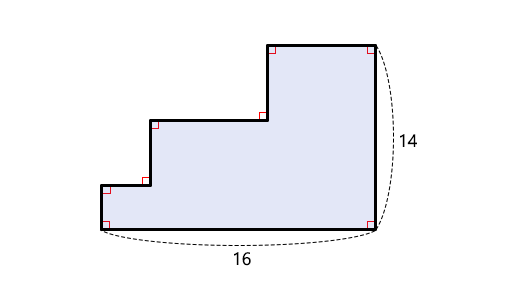The shape above is made of rectangles. If the length of the bottom side is 16 and the length of the right side is 14, what is the perimeter of the shape?

×